#Function Repository Resource:

# QuadraticFormDistribution

Represent the distribution of a quadratic form with a multivariate normal

Contributed by: Wolfram Research
 ResourceFunction["QuadraticFormDistribution"][{a,b,c},{μ,Σ}] represents the distribution of a quadratic form z.a.z + b.z + c for multivariate normal z.

## Details and Options

ResourceFunction["QuadraticFormDistribution"] is the distribution of z.a.z + b.z + c for real-valued p×p symmetric positive definite matrix a, length-p vector b, scalar c, and p-dimensional multivariate normal vector z.
QuadraticFormDistribution can be used with such functions as Mean and RandomReal.

## Examples

### Basic Examples

The mean of a quadratic form distribution:

 In:=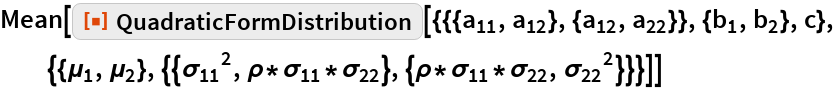Out=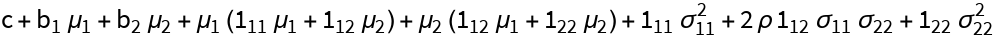The variance of a quadratic form distribution:

 In:=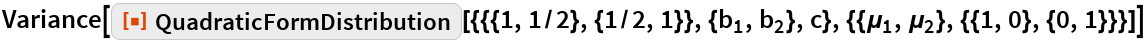Out=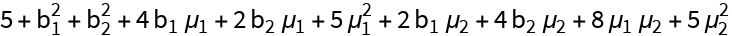### Scope

Generate a set of pseudorandom numbers that follow a quadratic form distribution:

 In:=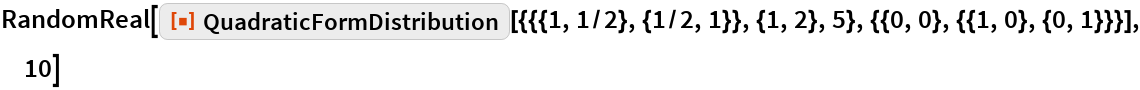Out=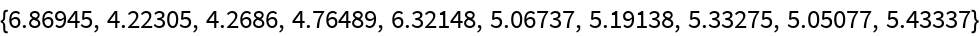The skewness and kurtosis of a quadratic form distribution:

 In:=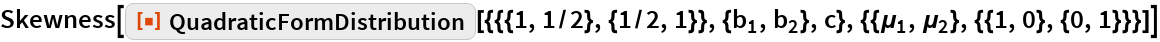Out=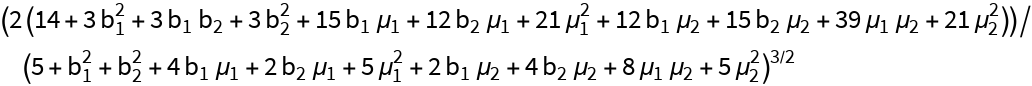In:=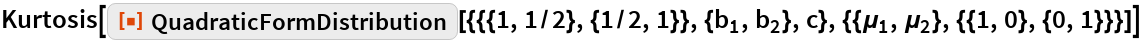Out=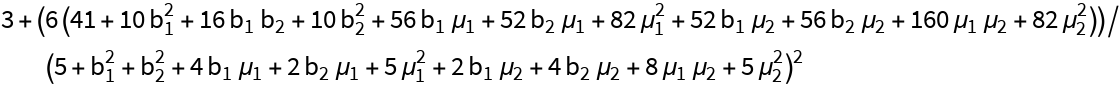### Possible Issues

PDF and CDF can only be evaluated using Series:

 In:=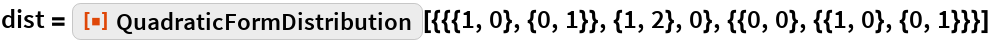Out=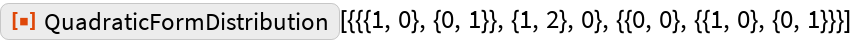In:=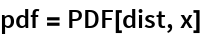Out=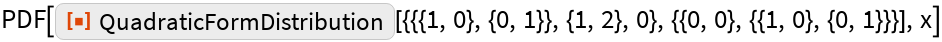In:=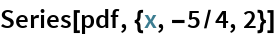Out=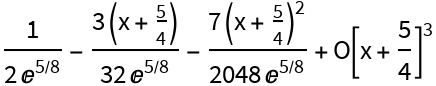In:=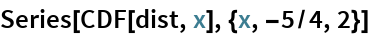Out=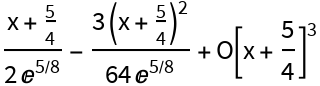Series expansion must be about the lower support point for the distribution:

 In:=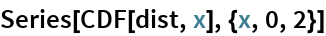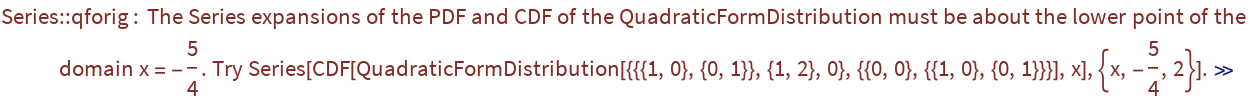Out=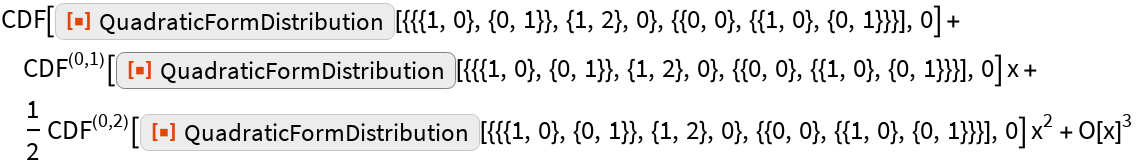Substitution of invalid parameters into symbolic outputs gives results that are not meaningful:

 In:=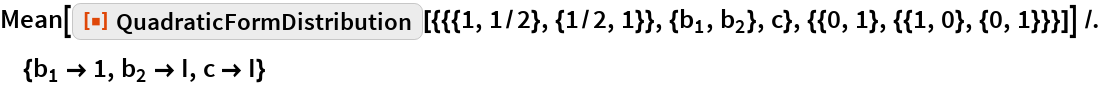Out=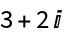## Requirements

Wolfram Language 11.3 (March 2018) or above